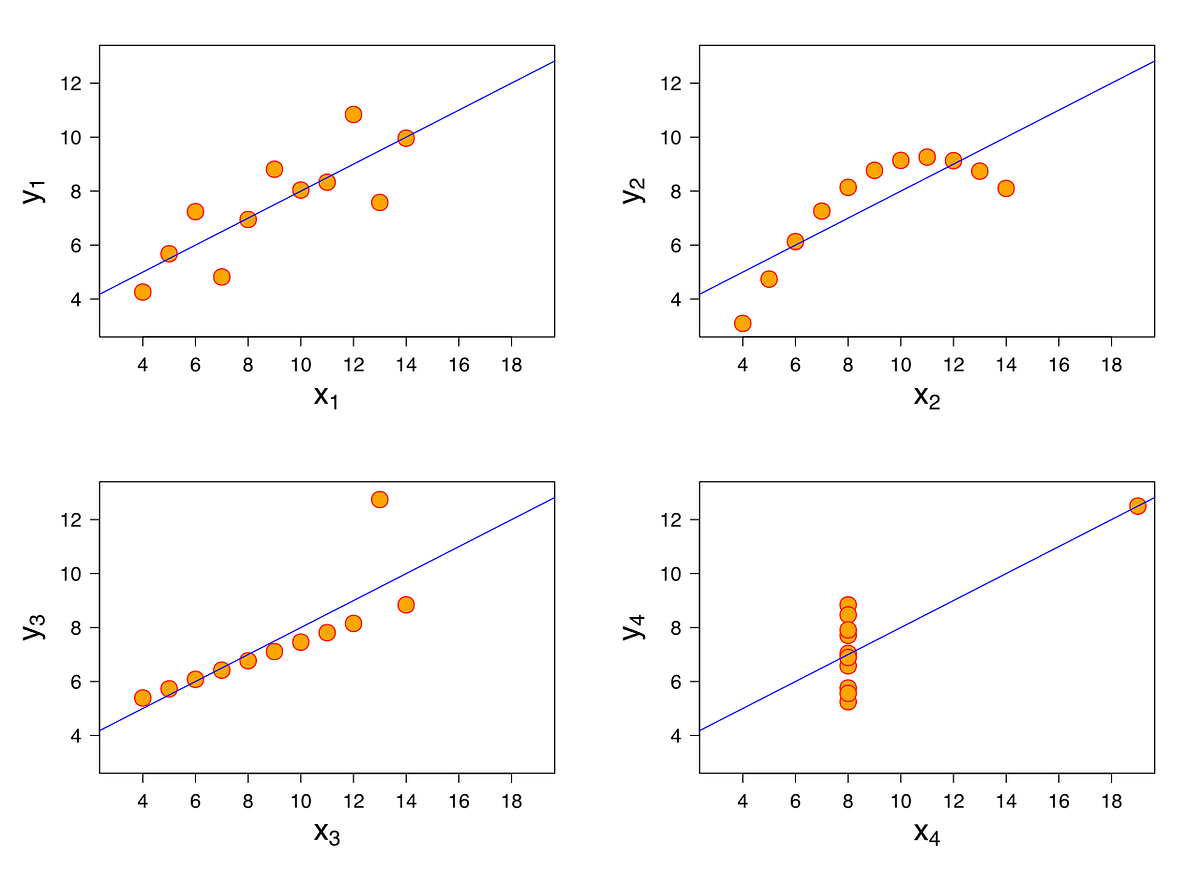# Linear Regression Model in Machine LearningLearning about the linear regression model in machine learning for predictive analysis . Linear regression is one of the most important regression models which are used in machine learning. In the regression model, the output variable, which has to be predicted, should be a continuous variable, such as predicting the weight of a person in a class.

Linear regression is one of the most important regression models which are used in machine learning. In the regression model, the output variable, which has to be predicted, should be a continuous variable, such as predicting the weight of a person in a class.

The regression model also follows the supervised learning method, which means that to build the model, we’ll use past data with labels, which helps predict the output variable in the future.

### Linear Regression

Using the linear regression model, we’ll predict the relationship between the two factors/ variables. The variable which we are expecting is called the dependent variable.

## Linear Regression VS Logistic Regression (MACHINE LEARNING)

Linear Regression VS Logistic Regression (MACHINE LEARNING). Linear Regression and Logistic Regression are two algorithms of machine learning and these are mostly used in the data science field.

## Regression: Linear Regression

Machine learning algorithms are not your regular algorithms that we may be used to because they are often described by a combination of some complex statistics and mathematics.

## What is Supervised Machine Learning

What is neuron analysis of a machine? Learn machine learning by designing Robotics algorithm. Click here for best machine learning course models with AI

## Pros and Cons of Machine Learning Language

AI, Machine learning, as its title defines, is involved as a process to make the machine operate a task automatically to know more join CETPA

## Simple Linear Regression Model using Python: Machine Learning

Learning how to build a simple linear regression model in machine learning using Jupyter notebook in Python. This article will see how we can build a linear regression model using Python in the Jupyter notebook.